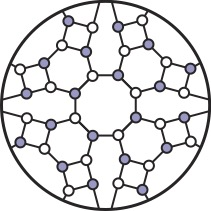## Mathematical Structures

Physics often advances when crisp mathematical structures are uncovered in a framework developed to describe observed phenomena. For example, in quantum field theory there is a vast discrepancy between the current calculational difficulty in making predictions for experiments and the simple, mathematical form of the end result. The Amplitudes program seeks to explain and exploit this surprising simplicity by reformulating the basic mathematical tools used to make predictions.

#### IGC members who study Mathematical Structures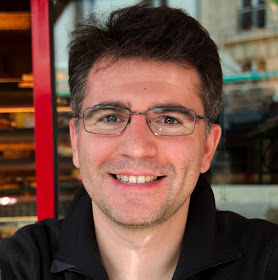Eugenio Bianchi Faculty Physics exb34@psu.edu +1 814 865 7533 315 Whitmore Laboratory CFT Quantum Universe, Mathematical Structures, Black Holes, Loop Quantum Gravity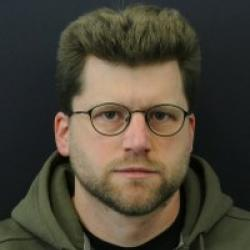Martin Bojowald Faculty Physics mob6@psu.edu +1 814 865 3502 304A Whitmore Laboratory CFT Mathematical Structures, Loop Quantum Gravity, Quantum Universe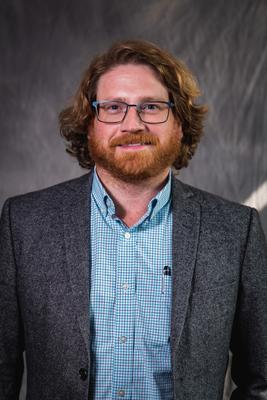Jacob Bourjaily Faculty Physics bourjaily@psu.edu +1 814 865 7533 321A Osmond Laboratory CFT Mathematical Structures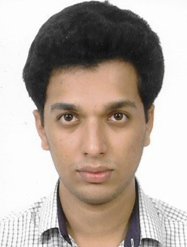Pranav Diwakar Graduate Student Physics pranavd@psu.edu +1 814 865 7533 204 Osmond Laboratory CFT Mathematical Structures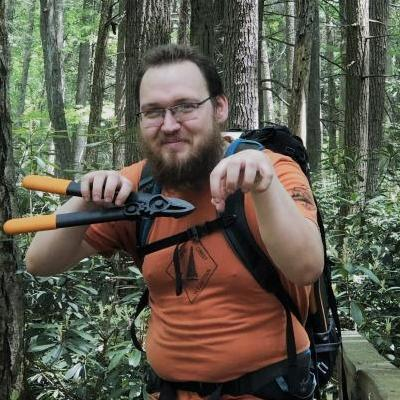Patrick Donaghue Graduate Student Math donaghue@psu.edu +1 814 865 7527 012 McAllister IGC Mathematical Structures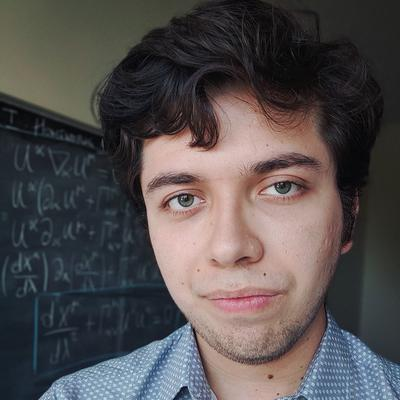Mauricio Gamonal Graduate Student Physics mfg5767@psu.edu -- 322 Whitmore Laboratory CFT Loop Quantum Gravity, Quantum Universe, Mathematical Structures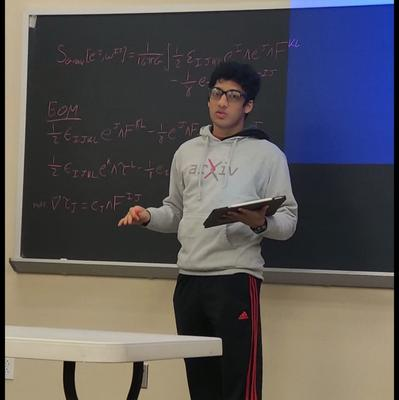Samarth Khandelwal Undergraduate Student Physics, Math smk6968@psu.edu N/A N/A Osmond Laboratory IGC, CFT Black Holes, Dynamic Universe, Gravitational Waves, Mathematical Structures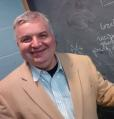Adrian Ocneanu Faculty Math axo2@psu.edu +1 814 865 4061 225 McAllister CFT Mathematical Structures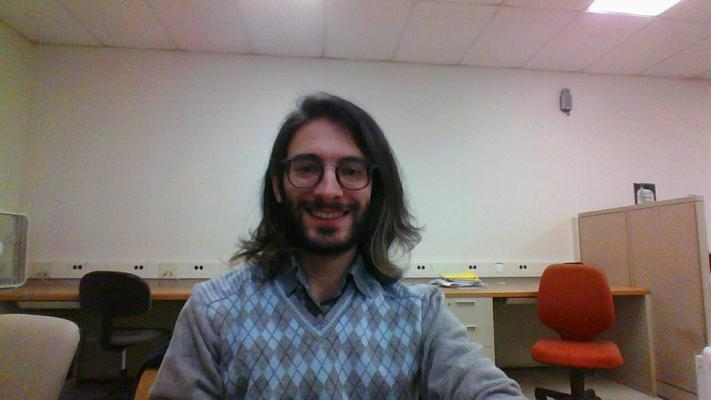Kokkimidis Patatoukos Graduate Student Physics kzp326@psu.edu 814 699 1747 005 Osmond Laboratory CFT Mathematical Structures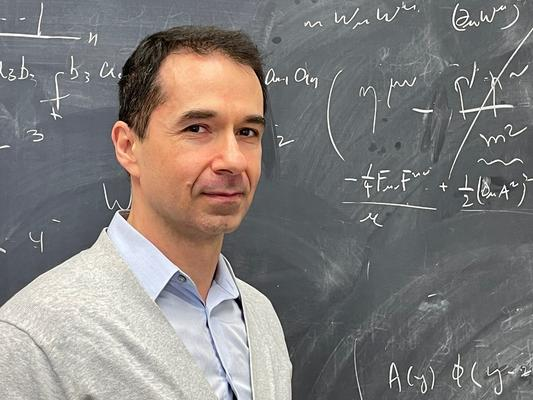Radu Roiban Faculty Physics rsr10@psu.edu +1 814 863 5811 320F Osmond Laboratory CFT Mathematical Structures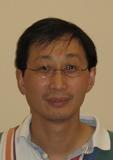Ping Xu Faculty Math pxx2@psu.edu +1 814 865 3517 109B McAllister CFT Mathematical StructuresYaqi Zhang Postdoc Physics yjz5289@psu.edu +1 814 865 7533 na Osmond Laboratory CFT Mathematical Structures